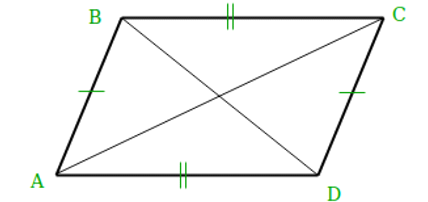# Find All Possible Coordinates of a Parallelogram in Java?

A parallelogram refers to the quadrilateral which has two pairs of parallel sides where opposite sides are equal in length, and the opposite angles are equal in measure.

In this article we are going to find all possible coordinates of a Parallelogram.

Basically, we will find all the possible coordinates from the given three coordinates to make a parallelogram of a non-zero area. Here the three given coordinates are not fixed points and can change.

Therefore, if three coordinates are given, we may claim that there are only three coordinates from which we can build a parallelogram.As per diagram given above, the opposite sides of a parallelogram are equal in length i.e., AD = BC and AB = CD, we can calculate the coordinates of the missing point (D) as

(Dx - Ax, Dy - Ay) = (Cx - Bx, Cy - By)

Dx = Ax + Cx - Bx

Dy = Ay + Cy - By

Let’s start!

## To show you some instances

### Instance-1

Suppose the three points are −

{a1 = 3, a2 = 2}, {b1 = 1, b2 = 0}, {c1 = 4, c2 = 2}

After finding all possible coordinates of a Parallelogram, result will be −

The (x, y) coordinates are −

0, 0

6, 4

2, 0

### Instance-2

Suppose the three points are −

{a1 = 7, a2 = 2}, {b1 = 3, b2 = 0}, {c1 =0, c2 = 1}

After finding all possible coordinates of a Parallelogram, result will be −

The (x,y) coordinates are −

10, 1

4, 3

-4, -1

### Algorithm

Step 1 − Declare the three coordinates of parallelogram.

Step 2 − Find the other possible coordinates using the formula.

Step 3 − Print the result.

### Multiple Approaches

We have provided the solution in different approaches.

• By Using Static Input Value

• By User Defined Method

Let’s see the program along with its output one by one.

## Approach-1: By Using Static Input Value

In this approach, we will take the three points as static input and apply the formula to print the result.

### Example

public class Main{

// main method
public static void main(String[] args){

//Declare the three coordinates of parallelogram
int a1 = 3, a2 = 2;
int b1 = 1, b2 = 0;
int c1 = 4, c2 = 2;

//find the other possible coordinates and printing it
System.out.println("The (x,y) coordinates are: ");
System.out.println(a1 + b1 - c1 + ", " + (a2 + b2 - c2));
System.out.println(a1 + c1 - b1 + ", " + (a2 + c2 - b2));
System.out.println(c1 + b1 - a1 + ", " + (c2 + b2 - a2));
}
}


### Output

The (x,y) coordinates are:
0, 0
6, 4
2, 0


## Approach-2: By Using User Defined Method

In this approach, first we will initialise a user defined method and we will take the three points as input and apply the formula to print the result.

### Example

public class Main {

// main method
public static void main(String[] s){
//Declare the three coordinates of parallelogram
int a1 = 7, a2 = 2;
int b1 = 3, b2 = 0;
int c1 = 0, c2 = 1;
//calling user defined function
func(a1, a2, b1, b2, c1, c2);
}

//user defined function
static void func(int a1, int a2, int b1, int b2, int c1, int c2){
//find the other possible coordinates and printing it
System.out.println("The (x,y) coordinates are: ");
System.out.println(a1 + b1 - c1 + ", " + (a2 + b2 - c2));
System.out.println(a1 + c1 - b1 + ", " + (a2 + c2 - b2));
System.out.println(c1 + b1 - a1 + ", " + (c2 + b2 - a2));
}
}


### Output

The (x,y) coordinates are:
10, 1
4, 3
-4, -1


In this article, we explored how to find all possible coordinates of a parallelogram in Java by using different approaches.